Thank you for visiting nature.com. You are using a browser version with limited support for CSS. To obtain the best experience, we recommend you use a more up to date browser (or turn off compatibility mode in Internet Explorer). In the meantime, to ensure continued support, we are displaying the site without styles and JavaScript.

# Spin hydrodynamic generation

## Abstract

Magnetohydrodynamic generation1,2,3,4 is the conversion of fluid kinetic energy into electricity. Such conversion, which has been applied to various types of electric power generation, is driven by the Lorentz force acting on charged particles and thus a magnetic field is necessary3,4. On the other hand, recent studies of spintronics5,6,7,8,9,10,11,12,13,14,15,16,17,18,19,20,21,22,23 have revealed the similarity between the function of a magnetic field and that of spin–orbit interactions in condensed matter. This suggests the existence of an undiscovered route to realize the conversion of fluid dynamics into electricity without using magnetic fields. Here we show electric voltage generation from fluid dynamics free from magnetic fields; we excited liquid-metal flows in a narrow channel and observed longitudinal voltage generation in the liquid. This voltage has nothing to do with electrification or thermoelectric effects, but turned out to follow a universal scaling rule based on a spin-mediated scenario. The result shows that the observed voltage is caused by spin-current6 generation from a fluid motion: spin hydrodynamic generation. The observed phenomenon allows us to make mechanical spin-current and electric generators, opening a door to fluid spintronics.

## Main

An electron is an elementary particle which carries internal angular momentum called an electron spin. The recent development of nanotechnology has enabled spins to be used to form a new field called spintronics. Various phenomena found in spintronics originate from angular-momentum exchange between the spin and other degrees of freedom, such as magnetization7,8 and light polarization9,10,11; so far, many degrees of freedom have been united into this angular-momentum exchange framework. However, the exchange has not been observed for the most common and ordinary carrier of angular momentum—namely, mechanical rotation of material objects.

The interaction which combines mechanical angular momentum and electron spin is called spin–rotation coupling12,13. With this coupling, mechanical rotation gives rise to spin voltages and spin currents. Here, the spin voltage is the driving force for a spin current, represented by μSμμ, where μ and μ respectively denote the electrochemical potential for spin-up and spin-down electrons16,17; a gradient of spin voltage drives a spin current.

To generate a gradient of spin voltage from mechanical motion, we have used flows of a liquid metal (Fig. 1a), as a gradient of mechanical rotation can easily be generated in a fluid. To describe spin voltage generation in a liquid, we expanded the angular-momentum conservation law for fluid dynamics to include angular-momentum transfer between a liquid and an electron spin. By introducing an antisymmetric stress tensor to the equation of motion for a liquid, the angular momentum of a fluid is predicted to be transferred into the electron spin (see Supplementary Information for details). The obtained equation for the spin voltage μS (the vector indicates a polarized direction) is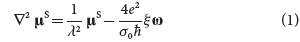where λ is the spin-diffusion length18 and ξ is related to the fluid viscosity caused by the angular-momentum transfer, which is added to the viscosity coefficient of a Newtonian fluid. The vorticity24ω ≡ rot v represents local mechanical rotation of a fluid, where v is the fluid velocity (Fig. 1b). Equation (1) implies that the vorticity acts as a spin-current source (Fig. 1c).

To detect spin voltage in a fluid, we have used the inverse spin Hall effect17,19,20,21,22 (ISHE). The ISHE converts a spin current into an electric field EISHE through the spin–orbit interaction of electrons. The spin current jS carries the spin σ. The relationship between EISHE and jS is given by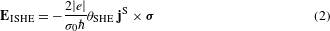where σ0 and θSHE are the electrical conductivity and the spin Hall angle, respectively. By measuring EISHE, the ISHE can be used to detect a spin current precisely (see Fig. 1d).

Figure 1e shows the geometry for mechanical spin-current generation in a cylindrical fluid channel used in the present study. In the channel, the vorticity ω is generated as a result of the viscosity near the inner wall and lies along the azimuthal direction (θ). Therefore, equations (1) and (2) predict a spatial gradient of μS along the radial direction (r) and the generation of EISHE due to the ISHE of a liquid metal along the axial direction (z).

Figure 2a shows a schematic illustration of the measurement set-up. In this set-up, we generate a flow of liquid metal mercury (Hg) in a cylindrical channel by applying a pulsed pressure ΔP. The channels consist of a quartz pipe and platinum (Pt) thin wires as electrodes embedded in the channel wall and electrically connected to the liquid metal via a pinhole to avoid disturbance of the fluid flow. The channel has to be an insulator for spin hydrodynamic (SHD) measurements to avoid thermoelectric effects between the channel and the liquid metal. The electrode material is chosen as Pt because of its weak chemical reactivity and its absolute thermopower being close to that of Hg; the observed relative Seebeck coefficient is SHg−Pt = + 0.06 μV K−1. The inner diameter and the length of the channel (the distance between the electrodes) are denoted as φ and L, respectively. To prevent it from charging up electrically, the Hg is connected to the ground at the inlet. We measured the electric voltage difference V between the ends of the channel filled with Hg. All the measurements were performed at room temperature.

Figure 2b shows the time evolution of V measured in the liquid Hg. The liquid flow in the channel starts at time t = 0 and ends at t = Δt. A clear V signal appears when Hg is flowing, as shown in Fig. 2b. The sign of V is reversed on reversing the flow direction. This unconventional voltage behaviour is the feature predicted for the ISHE induced by a mechanical motion described above: the direction of EISHE is predicted to be reversed by reversing the ω direction or the Hg flow direction (see Fig. 1e and equation (2)).

To further examine the voltage generation, we measured V for various values of mean flow velocity, v, and for several channels with different φ and L values. v is modulated by changing ΔP; the measurement result, shown in Fig. 2c, is reproduced well by the relation for a turbulent flow in a pipe24, being consistent with the fact that its Reynolds number,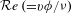, satisfies the turbulent flow condition,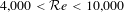, where ν is the kinetic viscosity. The time evolution of V shown in Fig. 2d clearly illustrates that the magnitude of V increases with increasing ΔP, or the mean flow velocity v. Figure 2e shows the friction velocity24 (v) dependence of V for channels with different φ and L values. The result shows that V increases with v but with different slopes for the different channels. Here, v is directly related to the ω gradient in a turbulent flow: the vorticity of a turbulent flow, ωθ(r), in a pipe is described24 by ωθ(r) = v2/ν in the region close to the wall, called the viscous sublayer, with ωθ(r) = v/[κ(r0r)] elsewhere. κ is the von Kármán constant and r0φ/2. The relationship between v and v for different φ is shown in the inset to Fig. 2f, which was calculated24 by averaging the velocity distribution of the turbulent flow in the pipe.

In this way, different channels were found to exhibit different V behaviour. However, we predict a scaling rule on the behaviour as follows. A spin voltage can be generated from ωθ(r) as a result of the spin–rotation coupling, and the spatial gradient of the spin voltage is then converted into spin currents in the liquid metal and into an electric voltage via the ISHE: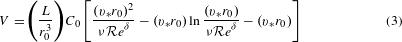where C0 ≡ (4|e|/κ)((θSHEλ2ξ)/σ0),(11.6 for the present study), and δ0 is the thickness of the viscous sublayer (see Supplementary Information for detailed derivation). This formula predicts that r03V/L exhibits a universal scaling with respect to vr0, identifying that the V signal is generated from the spin current.

In Fig. 2f, we replot all the data from Fig. 2e for different channels into a r03V/L versus vr0 scale. In this plot, surprisingly, all the different data collapse onto a single curve. This universal scaling behaviour is the very feature predicted above equation (3), demonstrating that the observed V signal is due to the ISHE induced by mechanically generated spin currents.

By fitting the data in Fig. 2f with equation (3), the parameter θSHEλ2ξ is estimated to be 5.9 × 10−25 J s m−1. If we assume typical magnitudes18,23 of θSHE and λ (θSHE 10−2 and λ 10−8 m), ξ is then estimated to be 6 × 10−7 J s m−3, or ξ/μ 4 × 10−4, where μ is the Newtonian viscosity. Here, we used parameters from ref. 24 for Hg: σ0 = 1.01 × 106 (Ω m)−1, ν = 1.2 × 10−7 m2 s−1 and μ = 1.6 × 10−3 J s m−3.

We also carried out measurements on Ga62In25Sn13(GaInSn), another chemically stable liquid metal (melting point: 5 °C), whose spin–orbit coupling may be weaker than that in Hg because all atoms comprising GaInSn are lighter than Hg. In the measurements, because an appropriate material is not available for the electrode in GaInSn, we used a special set-up, called a triangle set-up; the details of the set-up are explained later. Figure 3a shows the ΔP dependence of v in GaInSn and Hg. The velocity of GaInSn is faster than that in Hg because of the density difference. Figure 3b, c shows v versus V plots of GaInSn and Hg, and the time evolution of V for various values of ΔP in GaInSn, respectively. The data show that V is slightly suppressed by substituting Hg for GaInSn. Equations (1) and (2) phenomenologically indicate that the conversion from the vorticity into the voltage depends on θSHE and λ. When spin–orbit coupling decreases, θSHE decreases whereas λ tends to increase. Therefore, the effects of spin–orbit coupling on V partially compensate, resulting in the weak material dependence obtained from the GaInSn and Hg measurements. If we assume θSHEλ2ξ for GaInSn to be 3.0 × 10−24 J s m−1, equation (3) reproduces the observed V values. Here, we used parameters from ref. 25 for GaInSn: σ0 = 3.1 × 106 (Ω m)−1 and ν = 2.98 × 10−7 m2 s−1.Figure 3: Experimental results for GaInSn and comparison of voltage with inner-wall material.

Now, we examine the possibilities of other mechanisms that might give rise to voltage signals in the present set-up. First, the magnetohydrodynamic effect1,2,3,4 (MHD), or the effect of the Lorentz force on a liquid-metal flow caused by environmental magnetic fields, was found to be negligible; the electromotive force caused by the MHD is perpendicular to the liquid flow, and thus the voltage caused by the MHD should not depend on the length of a channel, which is contradictory to the observed signal. Also, we confirmed that the observed signal is not affected by the direction of the geomagnetic field.

Next, we examine contact electrification effects26,27,28,29, or the influence of charging effects of Hg due to electrochemical interaction with a channel wall, even though the Hg is connected to the ground. A flow of charged Hg might generate electric voltage in itself. The charging effect is known to depend on the material species26,27,28 and roughness29 of the contact surface. To change the property of the contact surface, we coated the inner wall of the channel with resin and measured V using the coated channel. Resin is known to exhibit different electrification properties from quartz26,27. The values of φ and L for the channel are 1.0 mm and 400 mm, respectively. The magnitude of the roughness was also changed approximately from 5 to 40 μm by the coating. As shown in Fig. 3d, the result indicates that the v dependence of V for the resin-coated channel is the same as that for the uncoated quartz channel, ruling out the contribution of the charging effect.

Finally, we examine the influence of thermoelectric effects. We constructed the special set-up shown in Fig. 4a. We call it the triangle set-up. This set-up allows us to completely eliminate the Seebeck voltage and to examine thermoelectric contamination in the SHD measurements. In the set-up, the voltage probes consist of Hg itself, allowing the voltage to be transferred to a common location for the attachment of wiring, as shown in Fig. 4a. Therefore, thermoelectric contamination is completely removed as follows. In Fig. 4b, we show the voltage signal generated in the triangle set-up filled with Hg, measured by applying a temperature difference ΔTH between the ends of the fluid channel, but without flowing Hg. The maximum magnitude of ΔTH is 20 K, much greater than the possible temperature difference in the liquid flow (approximately 0.1 K; see experimental results in Supplementary Information). Note, however, that no noticeable thermoelectric voltage is observed in going from a small to a large ΔTH, which shows that the thermoelectric effect is completely excluded in measuring the SHD signal. In fact, as shown in Fig. 4c, d, we observed flow-induced voltage signals in the triangle set-up, similar to those in the set-up shown in Fig. 2a (the simple set-up). Compared to this flow-induced signal in the triangle set-up, the thermoelectric signal in Fig. 4b is negligibly small even in the presence of a large temperature difference, proving that both the set-ups work perfectly to measure the SHD signal. To confirm this we compared the flow-induced voltage signal in the triangle set-up shown in Fig. 4c and that in the simple set-up shown in Fig. 4d; they are similar in terms of the flow velocity dependence and the signal magnitude. All the results indicate that the voltage signal generated in the liquid flow is completely unrelated to thermoelectricity.

From the point of view of applications, the observed generation effects can be used to make an electric generator and a spin generator without using magnets. It is thus certainly worthwhile to explore the effect in various liquid metals. We anticipate that the observed phenomena will bridge the gap between spintronics and hydrodynamics.

## References

1. Rosa, R. J. Magnetohydrodynamic Energy Conversion Ch. 4 (McGraw-Hill, 1968).

2. Alfvén, H. Existence of electromagnetic-hydrodynamic waves. Nature 150, 405–406 (1942).

3. Michiyoshi, I. & Nakura, S. Pressure drop of liquid mercury MHD power generators. J. Nucl. Sci. Technol. 9, 490–496 (1972).

4. Yamaguchi, H., Niu, X.-D. & Zhang, X.-R. Investigation on a low-melting-point gallium alloy MHD power generator. Int. J. Energy Res. 35, 209–220 (2011).

5. Maekawa, S. (ed.) Concepts in Spin Electronics (Oxford Univ. Press, 2006).

6. Maekawa, S., Valenzuela, S. O., Saitoh, E. & Kimura, T. (eds) Spin Current (Oxford Univ. Press, 2012).

7. Slonczewski, J. C. Current-driven excitation of magnetic multilayers. J. Magn. Magn. Mater. 159, L1–L7 (1996).

8. Berger, L. Emission of spin waves by a magnetic multilayer traversed by a current. Phys. Rev. B 54, 9353–9358 (1996).

9. Ando, K. et al. Photoinduced inverse spin-Hall effect: Conversion of light-polarization information into electric voltage. Appl. Phys. Lett. 96, 082502 (2010).

10. Kimel, A. V. et al. Ultrafast non-thermal control of magnetization by instantaneous photomagnetic pulses. Nature 435, 655–657 (2005).

11. Gøthgen, C., Oszwałdowski, R., Petrou, A. & Žutić, I. Analytical model of spin-polarized semiconductor lasers. Appl. Phys. Lett. 93, 042513 (2008).

12. Matsuo, M., Ieda, J., Saitoh, E. & Maekawa, S. Effects of mechanical rotation on spin currents. Phys. Rev. Lett. 106, 076601 (2011).

13. Matsuo, M., Ieda, J., Harii, K., Saitoh, E. & Maekawa, S. Mechanical generation of spin current by spin-rotation coupling. Phys. Rev. B 87, 180402 (2013).

14. Uchida, K. et al. Long-range spin Seebeck effect and acoustic spin pumping. Nature Mater. 10, 737–741 (2011).

15. Uchida, K., An, T., Kajiwara, Y., Toda, M. & Saitoh, E. Surface-acoustic-wave-driven spin pumping in Y3Fe5O12/Pt hybrid structure. Appl. Phys. Lett. 99, 212501 (2011).

16. Valet, T. & Fert, A. Theory of the perpendicular magnetoresistance in magnetic multilayers. Phys. Rev. B 48, 7099–7113 (1993).

17. Takahashi, S. & Maekawa, S. Spin current in metals and superconductors. J. Phys. Soc. Jpn 77, 031009 (2008).

18. Bass, J. & Pratt, W. P. Jr Spin-diffusion lengths in metals and alloys, and spin-flipping at metal/metal interfaces: An experimentalist’s critical review. J. Phys. Condens. Matter 19, 183201 (2007).

19. Saitoh, E., Ueda, M., Miyajima, H. & Tatara, G. Conversion of spin current into charge current at room temperature: Inverse spin-Hall effect. Appl. Phys. Lett. 88, 182509 (2006).

20. Valenzuela, S. O. & Tinkham, M. Direct electronic measurement of the spin Hall effect. Nature 442, 176–179 (2006).

21. Kimura, T., Otani, Y., Sato, T., Takahashi, S. & Maekawa, S. Room-temperature reversible spin Hall effect. Phys. Rev. Lett. 98, 156601 (2007).

22. Azevedo, A., Vilela Leaõ, L. H., Rodriguez-Suarez, R. L., Oliveira, A. B. & Rezende, S. M. dc effect in ferromagnetic resonance: Evidence of the spin-pumping effect? J. Appl. Phys. 97, 10C715 (2005).

23. Ando, K. et al. Inverse spin-Hall effect induced by spin pumping in metallic system. J. Appl. Phys. 109, 103913 (2011).

24. Landau, L. D. & Lifshitz, E. M. Fluid Mechanics 2nd edn, Ch. 1, 2 & 4 (Butterworth-Heinemann, 1987).

25. Morley, N. B., Burris, J., Cadwallader, L. C. & Nornberg, M. D. GaInSn usage in the research laboratory. Rev. Sci. Instrum. 79, 056107 (2008).

26. Shaw, P. E. Experiments on tribo-electricity. I. The tribo-electric series. Proc. R. Soc. Lond. A 94, 16–33 (1917).

27. Diaz, A. F. & Felix-Navarro, R. M. A semi-quantitative tribo-electric series for polymeric materials: The influence of chemical structure and properties. J. Electrostat. 62, 277–290 (2004).

28. Yarnold, G. D. The electrification of mercury indexes in their passage through tubes. Proc. Phys. Soc. 52, 196–201 (1940).

29. Hori, Y. & Saito, K. Effect of the surface roughness on contact charging of polypropylene with mercury. J. Inst. Electrostat. Jpn 24, 42–46 (2000).

## Acknowledgements

The authors thank Y. Fujikawa, R. Iguchi, Y. Ohnuma, J. Ohe, R. Haruki, Y. Shiomi, K. Ando, M. Mizuguchi and T. Seki for valuable discussions. This work was supported by ERATO-JST ‘Spin Quantum Rectification Project’, Japan, a Grant-in-Aid for Scientific Research on Innovative Areas from MEXT, Japan, a Grant-in-Aid for JSPS Fellows from JSPS, Japan, a Grant-in-Aid for Young Scientists B (24740247 and 24760722) from MEXT, Japan, a Grant-in-Aid for Challenging Exploratory Research (26610108) from MEXT, Japan, a Grant-in-Aid for Scientific Research A (24244051 and 26247063) from MEXT, Japan, and a Grant-in-Aid for Scientific Research C (25400337 and 15K05153) from MEXT, Japan.

## Author information

Authors

### Contributions

R.T., M.O., K.H., H.C. and E.S. designed the experiments; R.T., M.O. and K.H. collected and analysed the data; S.O. supported the experiments; R.T., K.H., M.M., J.I. and S.T. developed the theoretical explanations; S.M. and E.S. planned and supervised the study; R.T., E.S., M.M. and J.I. wrote the manuscript; J.I. and E.S. coined the term ‘spin hydrodynamic (SHD) generation’. All authors discussed the results and commented on the manuscript.

### Corresponding authors

Correspondence to R. Takahashi or E. Saitoh.

## Ethics declarations

### Competing interests

The authors declare no competing financial interests.

## Supplementary information

### Supplementary information

Supplementary information (PDF 823 kb)

## Rights and permissions

Reprints and Permissions

• ### Chiral magnetic effect reveals the topology of gauge fields in heavy-ion collisions

• Dmitri E. Kharzeev
• Jinfeng Liao

Nature Reviews Physics (2021)

• ### Topological or rotational non-Abelian gauge fields from Einstein-Skyrme holography

• Casey Cartwright
• Benjamin Harms
• Matthias Kaminski

Journal of High Energy Physics (2021)

• ### Generation of Effective Field Gradient and Spin Current by a Flow of Liquid Helium-3

• Y. Tsutsumi
• S. Maekawa

Journal of Low Temperature Physics (2021)

• ### Spintronic devices for energy-efficient data storage and energy harvesting

• Jorge Puebla
• Junyeon Kim
• Yoshichika Otani

Communications Materials (2020)

• ### Giant spin hydrodynamic generation in laminar flow

• R. Takahashi
• H. Chudo
• E. Saitoh

Nature Communications (2020)

## Search